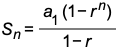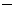PROOFS OF SOME FORMULAS USED FOR GEOMETRIC SEQUENCES AND SERIESFINDING THE GENERAL TERM OF A GEOMETRIC SEQUENCE an= a1r n - 1

Consider a geometric sequence whose first term is a1 and whose common ratio is r. Let's begin by writing the first six terms.

a1                    a1r        a1r 2      a1r 3      a1r 4      a1r 5

a1                     a2           a3         a4         a5         a6

Compare each power of r and the associated subscript of a. The power of r is 1 less than the associated subscript of a.

Therefore, the formula for the nth term must be

an= a1r n - 1

which is the general term of a geometric sequence.

FINDING THE SUM OF N-TERMS OF A GEOMETRIC SEQUENCESn = a1  + a1r  + a1r 2 + ... + a1r n - 2  +  a1r n - 1

Now, multiply both sides of the equation by r:

rSn = a1r  + a1r 2  + a1r 3 + ... + a1r n - 1  +  a1r n

We will now subtract the second equation for the first equation to get

SnrSn = a1a1r n

We are now going to factor out Sn on the left and a1 on the right to get

Sn(1r) = a1 (1r n)

Finally, we will solve for Sn to getwhich is the sum of the first n terms of a geometric sequence.

FINDING THE SUM OF THE TERMS OF AN INFINITE GEOMETRIC SEQUENCEWe will first investigate what happens to r n in the formulaif n is allowed to get larger and larger.We must understand that the term r n will get smaller and smaller and will approaches 0, BUT only as long as r is any number between - 1 and 1.

For example, observes what happens to r n when n gets larger and larger given.We see that r n gets smaller and smaller ultimately approaching 0.

So let us replace r n with 0 in the formula. We will get. Note that we no longer write Sn because there is no n in the formula!is the formula for the sum of the terms of an infinite geometric sequence AS LONG AS r is between1 and 1.

If r is greater than or equal to 1, there is no sum of the infinite geometric squence.